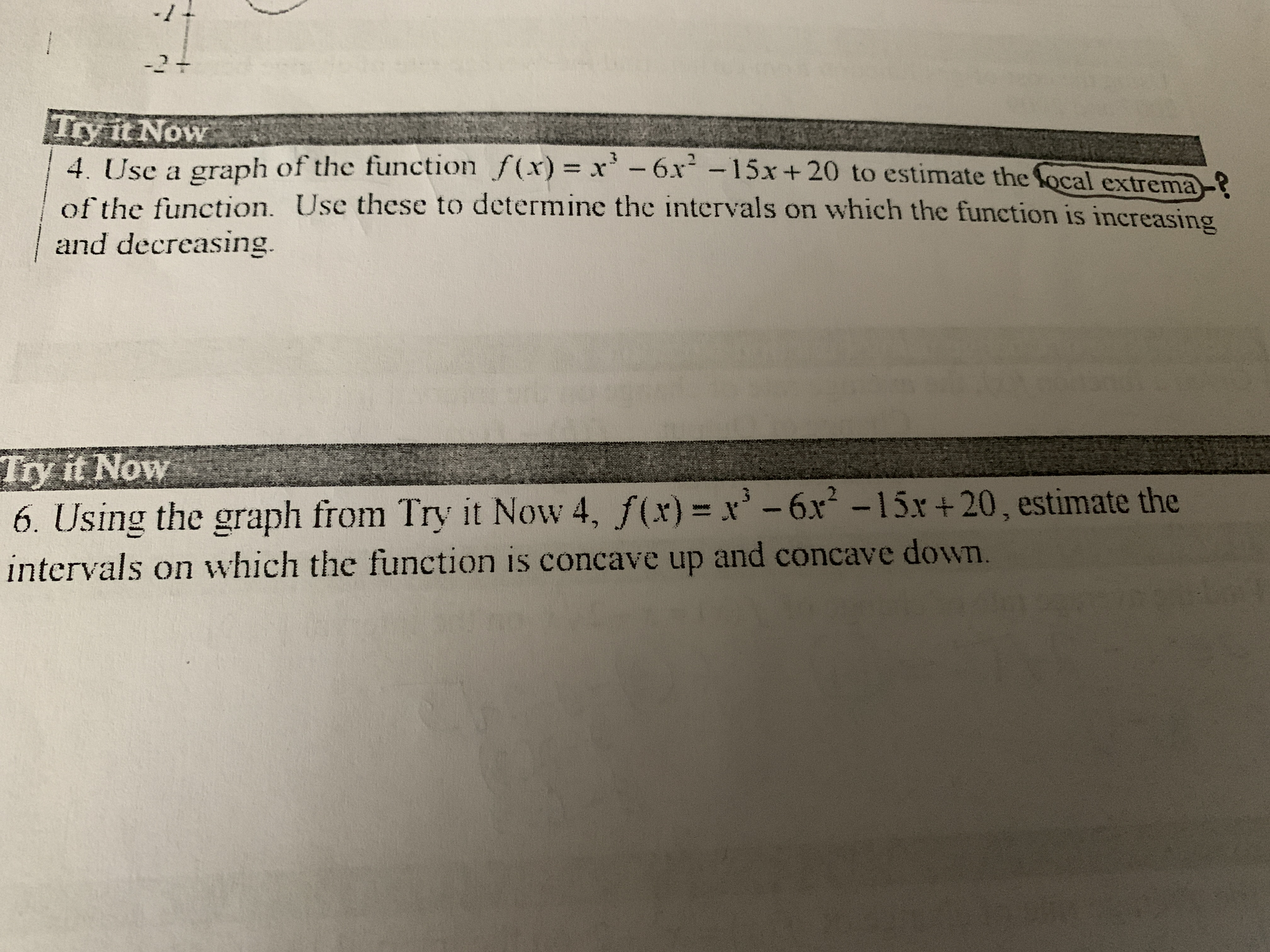# -11-2+Try it Now4. Use a graph of the function f(x)= x -6x-15x + 20 to estimate the focal extrema?of the function. Use these to determine the intervals on which the function is increasingand decreasing.Try it Now6. Using the graph from Try it Now 4, f(x)= x-6x -15x+20, estimate theintervals on which the function is concave up and concave down.

Question
4 views

I am 16 years old and dropped of high school to get a ged and go to college. These dudes put me in a precalculus class. The last math class i took was geometry. I am struggling on the first day and its getting worse. My second day is today and I have no idea how to graph these complex functions. I downloded desmos to graph the funtions but I am still lost. Help.help_outlineImage Transcriptionclose-11 -2+ Try it Now 4. Use a graph of the function f(x)= x -6x-15x + 20 to estimate the focal extrema? of the function. Use these to determine the intervals on which the function is increasing and decreasing. Try it Now 6. Using the graph from Try it Now 4, f(x)= x-6x -15x+20, estimate the intervals on which the function is concave up and concave down. fullscreen
check_circle

star
star
star
star
star
1 Rating
Step 1

Local extrema’s in a graph, are the points where, a tangent at that point will be parallel to x-axis. In other words, we can say, that the slope at the point is zero.

Step 2

Now, how do we find the points where the slope is zero in a graph?

Mathematically, we differentiate the equation of the graph and equate it to zero.

Graphically, it will be the top or bottom of the wave where the graphs turn. Like the points P and Q  as shown below for the function f(x) = x3-6x2-15x+20.

Step 3

Also, from the graph we can find the co-ordinate of...

### Want to see the full answer?

See Solution

#### Want to see this answer and more?

Solutions are written by subject experts who are available 24/7. Questions are typically answered within 1 hour.*

See Solution
*Response times may vary by subject and question.
Tagged in
MathCalculus

### Derivative